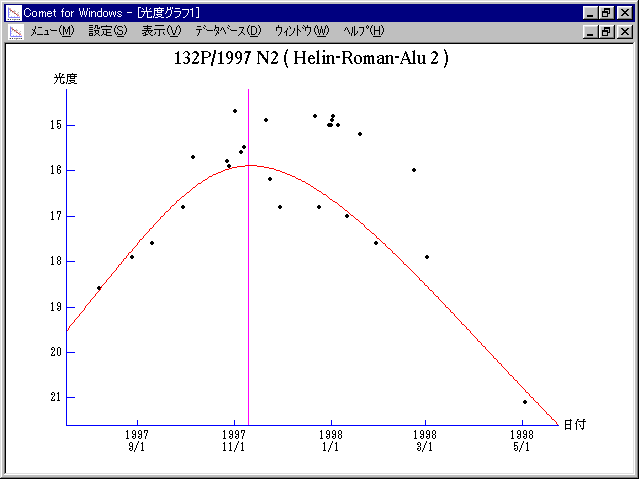# \$B%X%j%s(B-\$B%m!<%^%s(B-\$B%"%k!

132P/Helin-Roman-Alu 2 (1997)###\$B%W%m%U%#!<%k(B

 \$BId9f(B 132P/1997 N2 \$B8!=PF|(B 1997\$BG/(B7\$B7n(B13\$BF|(B \$B8!=P8wEY(B 19.6\$BEy(B \$B8!=P C. W. Hergenrother (Mt. Hopkins)

###\$B50F;MWAG(B

```   The following improved orbital elements by Kenji Muraoka, are
from 114 observations 1989 Oct. 26 to 1998 Mar. 9, perturbations
by 9 Plantes, Moon and 5 minor planets were taken into account.
The mean residual is +/- 0.64 arc seconds.

Epoch  =  1997 Nov.  8.0  TT       JDT = 2450760.5
T  =  1997 Nov. 10.09264       +/- 0.00023 (m.e.) TT
Peri. =  220.95870                +/- 0.00020
Node  =  178.47974                +/- 0.00030   (2000.0)
Incl. =    5.77564                +/- 0.00003
q  =    1.9100178              +/- 0.0000031 AU
e  =    0.5316521              +/- 0.0000007
a  =    4.0782030              +/- 0.0000023 AU
n  =    0.11967428             +/- 0.00000010
P  =    8.236                  +/- 0.0000069  years
```

###\$B@1?^(B1997\$BG/(B 6\$B7n(B 1\$BF|!A(B1998\$BG/(B 7\$B7n(B 6\$BF|(B

###\$B8wEYJQ2=(B

```        m1 = 7.2 + 5 log\$B&\$(B + 31 log r(t - 26)
```##### \$B50F;MWAG\$OB<2,7r<#;a\$N7W;;\$K\$h\$k\$b\$N\$G\$9!#(B \$B@1?^\$O(B StellaNavigator Ver.2.0 for Windows (\$B%"%9%H%m%"!<%D(B \$BJTCx(B / \$B%"%9%-!<=PHG6I4)(B) \$B\$G:n@.\$7\$?\$b\$N\$G\$9!#(B \$B8wEY%0%i%U\$O(BComet for Windows\$B\$G:n@.\$7\$?\$b\$N\$G\$9!#(B